## Python学习笔记（）——Numpy

2023/11/30 10:16:57

# Numpy

NumPy Numerical Python 的简称）是高性能科学计算和数据分析的基础包，

## NumPy库主要功能

ndarray( 数组 ) 是具有矢量算术运算和复杂广播能力的多维数组。

## NumPy库的安装

CMD
# 打开命令窗口
pip install numpy # cmd 中输入

## NumPy库的调用

import numpy as np #约定俗成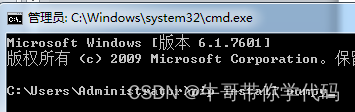## 多维数据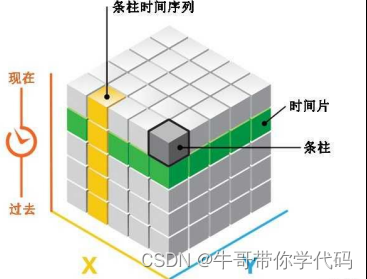[3.13, 4.13, 2,34] 有序
{3.13, 4.13, 2,34} 无序

### 举例：

a=[0,1,2,3,4]
b=[5,6,7,8,9]
c=[]
for i in range(0,5):
c.append(a[i]**2+b[i]**3)
import numpy as np
a_array=np.array(a)
b_array=np.array(b)
c=a_array**2+b_array**3

## 数组

NumPy 库处理的最基础数据类型是由同种元

numpy 数组的维数称为秩，每一个线性的数组称为轴。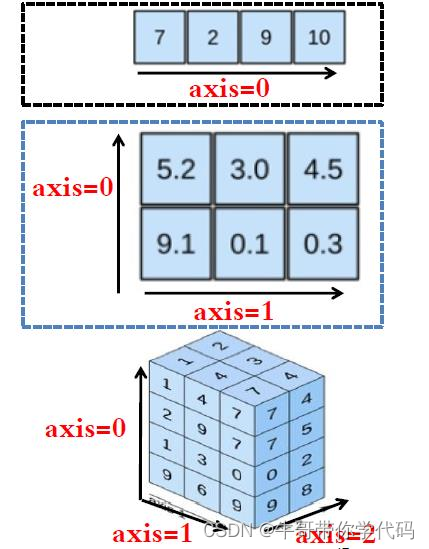### 例子

ndarray 在程序中的别名是： array.
np.array() 输出成 [] 形式，元素由空格分割 .
``````>>> import numpy as np
>>> a=[[1,2],[3,4]]
>>> a_array=np.array(a)
>>> a_array
array([[1, 2],
[3, 4]])
>>> type(a_array)
numpy.ndarray``````

## 数组创建

Python 中的列表 、元组等类型创建 ndarray 数组

## 内置数据结构创建数组

np.array(list/tuple, dtype=np.float32)

例子

``````>>> np.array([[1,2],[3,4]])
>>> np.array(((1,2),(3,4)))
array([[1, 2],
[3, 4]])``````

``````>>> np.array([[1,1.1],(2,2.1)])
array([[1. , 1.1],
[2. , 2.1]])``````

## 数组元素的类型

dtype( 数据类型 ) 是一个特殊对象，它含有 ndarry 将一块内存解释为特定数 据类型所需要的信息。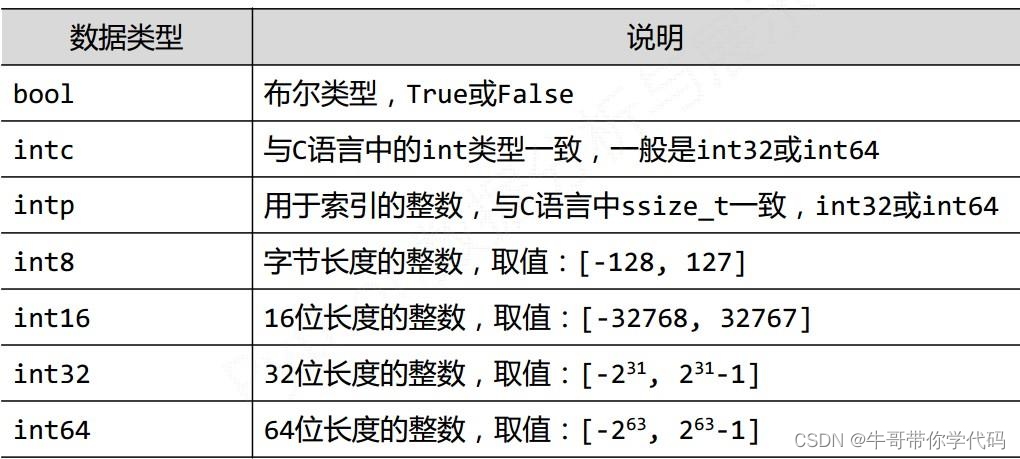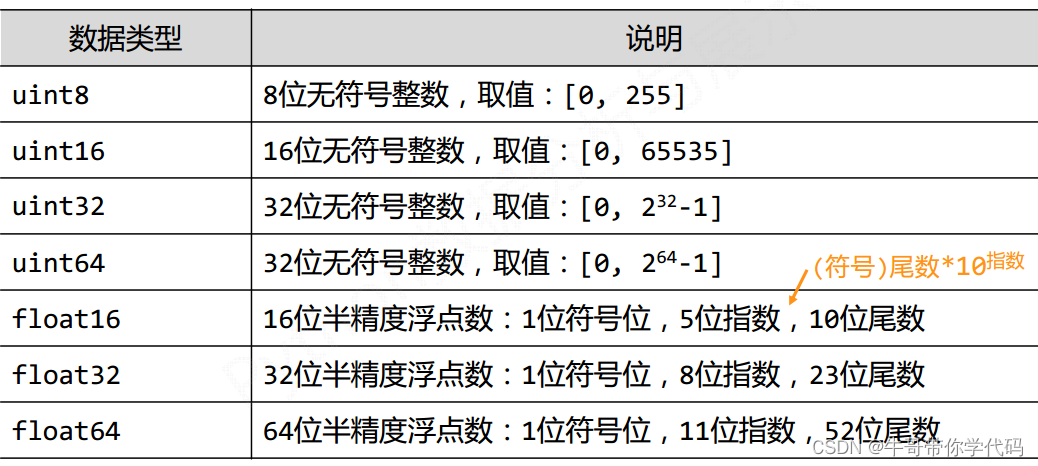ndarray 为什么要支持这么多种元素类型？
Python语法仅支持整数、浮点数和复数 3 种类型
Ndarry 的优势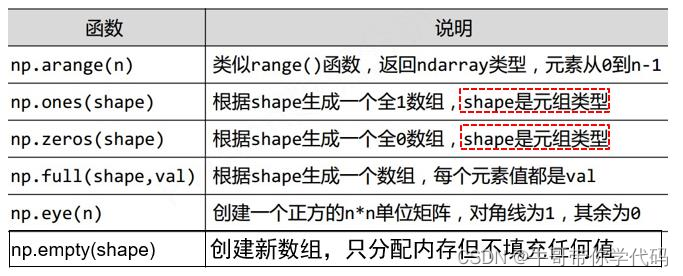``````>>> np.arange(10)
array([0, 1, 2, 3, 4, 5, 6, 7, 8, 9])

>>> np.ones((3,6))
array([[1., 1., 1., 1., 1., 1.],
[1., 1., 1., 1., 1., 1.],
[1., 1., 1., 1., 1., 1.]])

>>> np.ones((2,4),dtype=np.int32) :
array([[1, 1, 1, 1],
[1, 1, 1, 1]])

>>> np.eye(4)
array([[1., 0., 0., 0.],
[0., 1., 0., 0.],
[0., 0., 1., 0.],
[0., 0., 0., 1.]])

>>> np.full((2,4),4)
array([[4, 4, 4, 4],
[4, 4, 4, 4]])

``````

## 已知尺度大小创建数组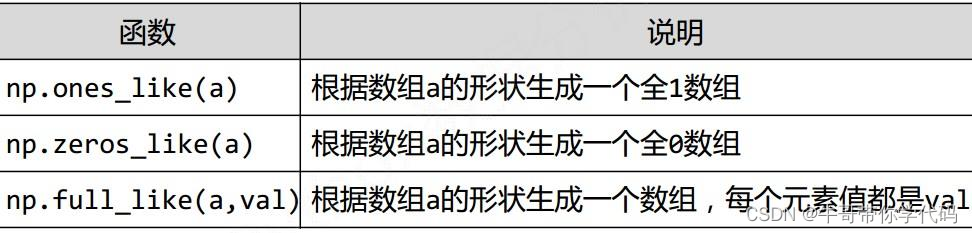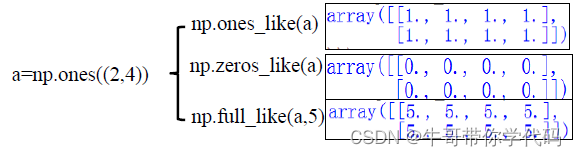### 数组对象的属性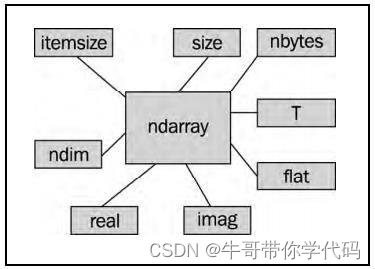``````a = np.array([[0, 1, 2, 3], [4, 5, 6, 7]]) #二维数组
b = np.array([0, 1, 2, 3]) #一维数组``````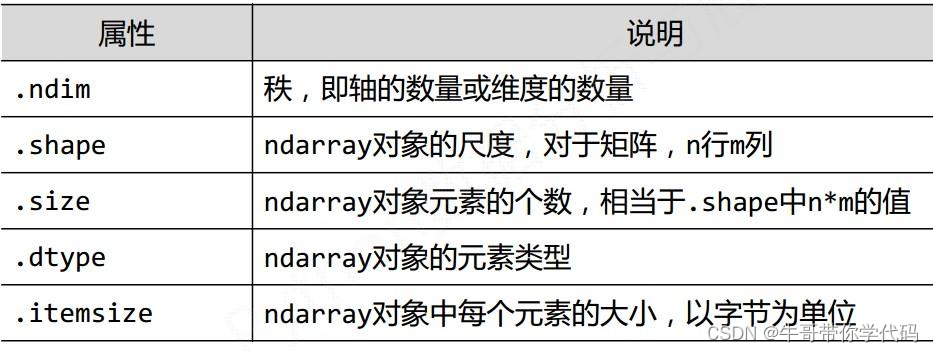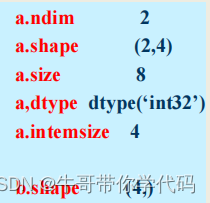## 维度的改变

对于创建后的ndarray数组，可以对其进行维度变换和元素类型变换。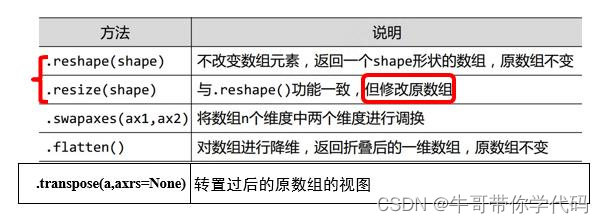``````>>> a = np.ones((2,3,4), dtype=np.int32)
>>> a.reshape((3,8))
array([[1, 1, 1, 1, 1, 1, 1, 1],
[1, 1, 1, 1, 1, 1, 1, 1],
[1, 1, 1, 1, 1, 1, 1, 1]])
>>> a #a的内容改变
array([[[1, 1, 1, 1],
[1, 1, 1, 1],
[1, 1, 1, 1]],
[[1, 1, 1, 1],
[1, 1, 1, 1],
[1, 1, 1, 1]]])``````
``````>>> a.flatten() #平铺
array([1, 1, 1, 1, 1, 1, 1, 1, 1, 1, 1, 1, 1,
1, 1, 1, 1, 1, 1, 1, 1, 1,1, 1])
>>> aa=np.arange(8).reshape((2,4))
array([[0, 1, 2, 3],
[4, 5, 6, 7]])
In : aa.transpose() #转置
array([[0, 4],
[1, 5],
[2, 6],
[3, 7]])``````
``````#不确定数目
>>> a.reshape((4,-1))
array([[1, 1, 1, 1, 1, 1],
[1, 1, 1, 1, 1, 1],
[1, 1, 1, 1, 1, 1],
[1, 1, 1, 1, 1, 1]])``````

## 一维数组转换为列表

array.tolist()
``````>>> np.arange(8).reshape((2,4))
array([[0, 1, 2, 3],
[4, 5, 6, 7]])``````
``````>>> np.arange(8).reshape((2,4)).tolist()
Out: [[0, 1, 2, 3], [4, 5, 6, 7]``````

## 二维数组：轴的概念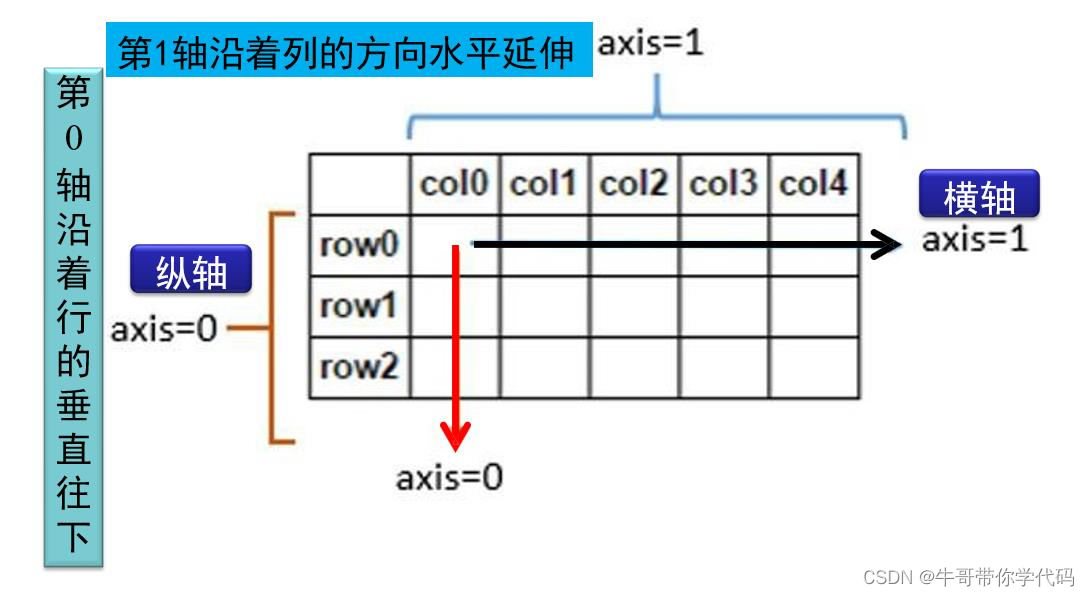## 二维数组：组合操作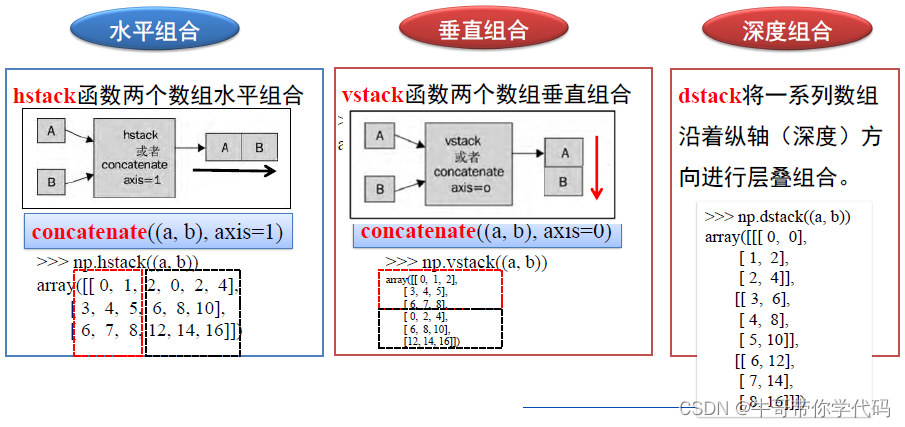## 二维数组：分割操作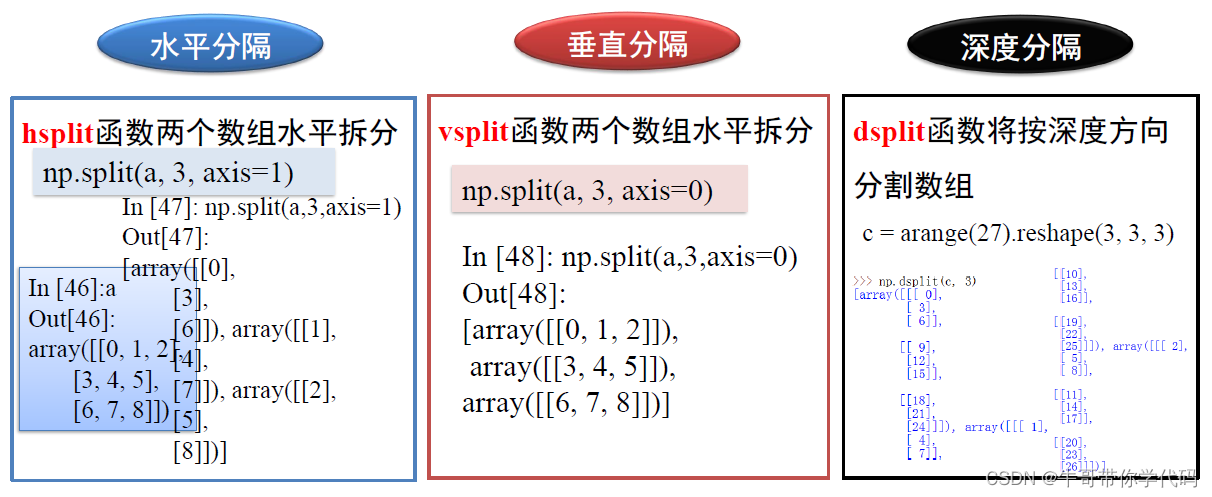``````>>> a=np.arange(9).reshape((3,3))
array([[0, 1, 2],
[3, 4, 5],
[6, 7, 8]]
>>> b=np.split(a, 3, axis=1)
[array([,
,
]),
array([,
,
]),
array([,
,
])]``````

# 数组的索引

## 一维数组的基本索引

``````>>> a=np.arange(1,6)
>>> a
array([1, 2, 3, 4, 5])``````
``````## 单个索引，切片索引
>>> a
3
>>> a[1:4:2]
array([2, 4])``````
``````## 索引值为序列
>>> a[1,3] #列表
array([2, 4])
>>> a[np.array([1,3])] #数组
array([2, 4])``````

## 可变对象——数组

``````>>> a=np.arange(1,6)
>>> b=a
>>> b[-1]=8
>>> b
array([1, 2, 3, 4, 8])
>>> a
array([1, 2, 3, 4, 8])
>>> b=a[:]
>>> b[-1]=8
>>> b
array([1, 2, 3, 4, 8])
>>> a
array([1, 2, 3, 4, 8])``````

## 数组切片与视图

``````>>> a=np.arange(1,6)
>>> b=a[1:3]
>>> b[-1]=8
>>> b
array([2, 8])
>>> a
array([1, 2, 8, 4, 5])``````
``````>>> b=a[1:3].copy()
>>> b[-1]=8
>>> a
array([1, 2, 8, 4, 5]) # 保持不变``````

## 二维数组的基本索引

0 索引值，轴 1 索引值。

ndarry [axis0_index,axis1_index]
``````>>> arr2d =np.arange(9).reshape(3,3)
>>> arr2d
array([[0, 1, 2],
[3, 4, 5],
[6, 7, 8]])
>>> arr2d[1,2]
5``````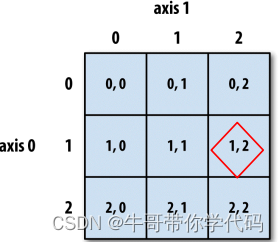ndarry[axis0_slice, axis1_slice]
``````>>> arr2d =np.arange(9).reshape(3,3)
>>> arr2d
array([[0, 1, 2],
[3, 4, 5],
[6, 7, 8]])
>>> arr2d[:2] #默认行索引
array([[0, 1, 2],
[3, 4, 5]])``````
``````>>> arr2d[:2, : ] #：表示所有元素
array([[0, 1, 2],
[3, 4, 5]])``````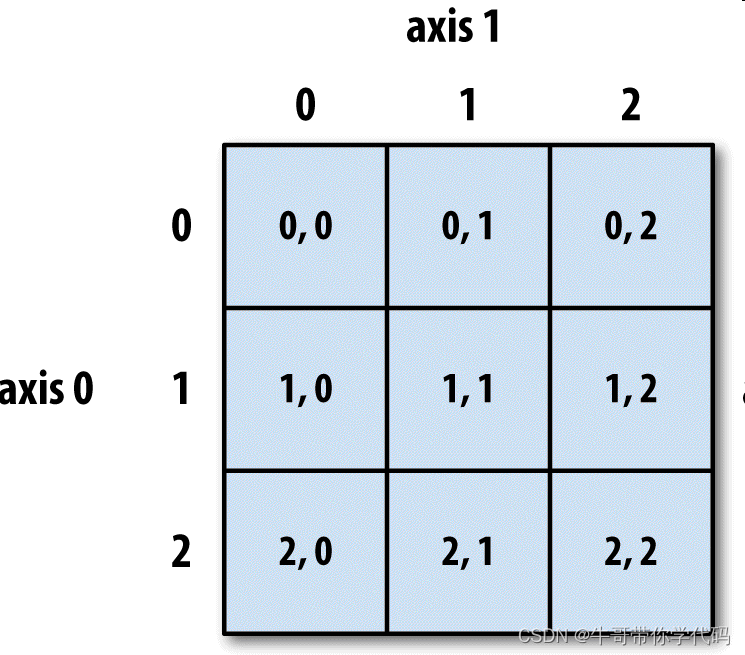## 二维数组的切片索引实例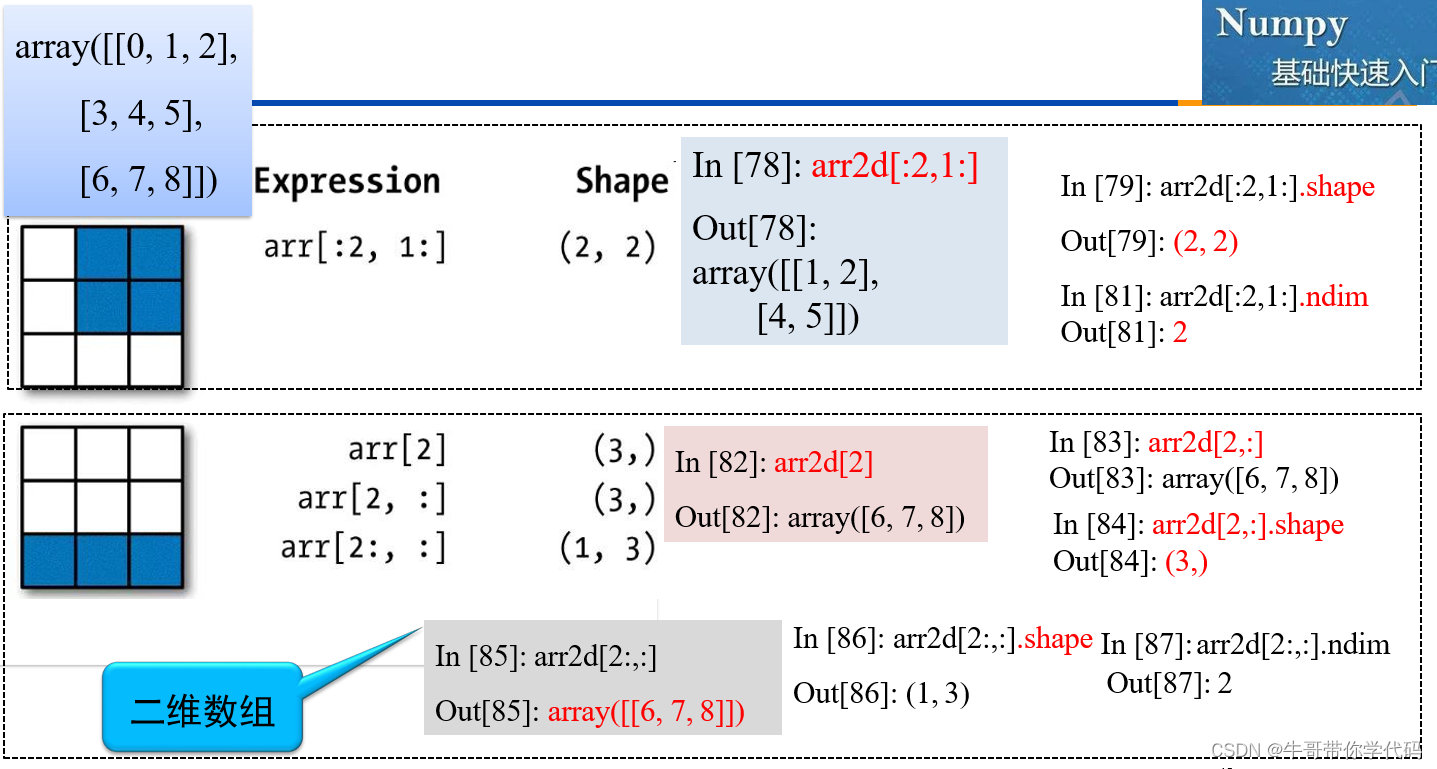## 二维数组增删改查操作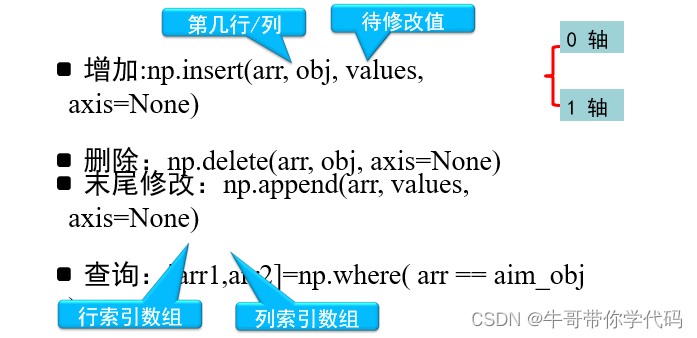实例

``````>>> np.insert(a,1,[0,0,0,0],0) #增加
array([[ 0,1, 2,3],
[0,0,0,0],
[4,5,6,7],
[8,9,10,11]])

>>> np.delete(a,0,1) #删除含有0的列
array([[ 1,2,3],
[ 5,6,7],
[ 9, 10, 11]])

>>> np.append(a, [[1,2,3,4]], 0)
#末尾修改
array([[ 0,1, 2,3],
[ 4,5,6,7],
[ 8,9,10,11],
[ 1,2,3,4]]) :

>>> np.where(a==1)#查找含有1的
(array(, dtype=int64),
array(, dtype=int64))``````

# 数组的矢量化

``````>>> a=np.arange(1,9).reshape((2,4))
>>> a
array([[1, 2, 3, 4],
[5, 6, 7, 8]])``````
``````>>> a**2
array([[ 1, 4, 9, 16],
[25, 36, 49, 64]], dtype=int32)
>>> 1/a
array([[1, 0.5, 0.333333,0.25],
[0.2 ,0.166667, 0.14285, 0.125])``````

# 布尔型索引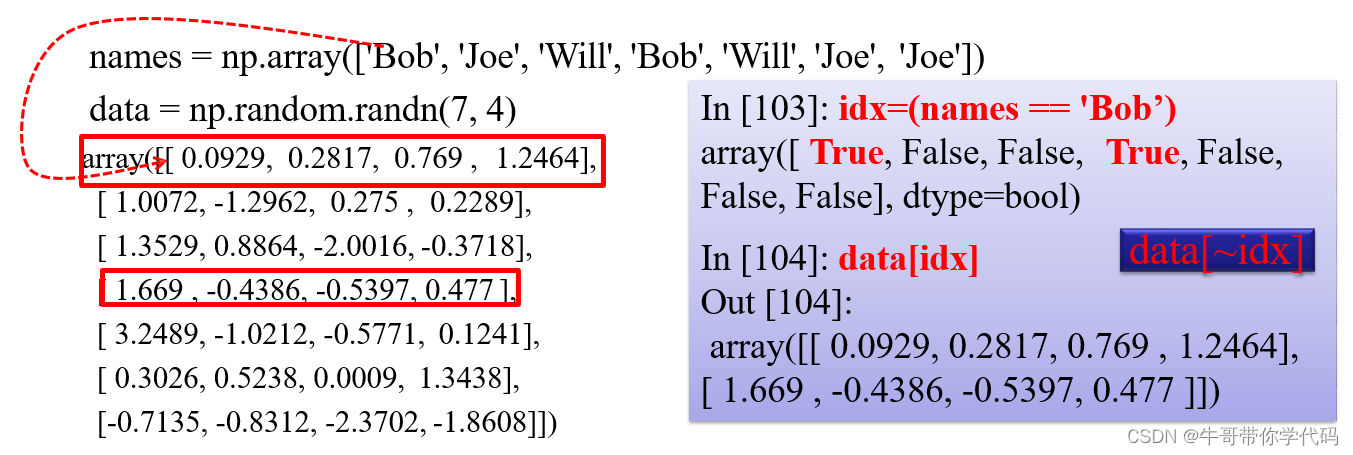布尔型索引：切边索引数组直接赋值

``````>>> data = np.random.randn(7, 4)
>>> a
array([[-1.04067731, -0.03879518, 0.98019589, -0.88795274],
[-1.18705612, 0.4635102 , 0.91812337, -0.81458408],
[ 0.86988195, 0.3959186 , 0.33063004, -0.1769584 ],
[-0.46452414, -0.46827261, -0.20851302, 0.22864186],
[-0.04973137, -0.23765974, 0.54263156, -0.06470696],
[-0.89274831, 2.5559152 , 1.02892789, 2.16099481],
[ 2.07400286, -1.96976106, -0.97007937, 1.84897368]])
array([[ True, True, False, True], [ True, False, False, True], [False,
False, False, True],
[ True, True, True, False], [ True, True, False, True], [ True, False,
False, False], [False, True, True, False]])

>>> idx=(data<0)
>>> data[data < 0] = 0
>>> data
array([[0., 0., 0.98019589, 0.],
[0., 0.4635102 , 0.91812337, 0.],
[0.86988195, 0.3959186 , 0.33063004, 0.],
[0., 0., 0., 0.22864186],
[0., 0., 0.54263156, 0.],
[0., 2.5559152 , 1.02892789, 2.16099481],
[2.07400286, 0., 0., 1.84897368]])``````

## 用于布尔型数组的方法

sum 经常被用来对布尔型数组中的 True 值计数。
any 用于测试数组中是否存在一个或多个 True
all 则检查数组中所有值是否都是 True
``````>>> arr = np.random.randn(10)
>>> arr
array([-0.71497659, -0.97902783, -
0.18698594, 0.08350516, -0.42339716,
-0.86465629, 0.70777104, -1.22436206, -
1.02389181, -1.30741898])
>>> arr_bool=(arr>=0)
array([False, False, False, True, False, False,
True, False, False, False])

>>> arr_bool.sum()
2 #大于0的个数
>>> arr_bool.any()
True #存在大于0
>>> arr_bool.all()
False #是否均大于0``````

## 一元算数函数

ndarray 中的数据执行元素级运算的函数。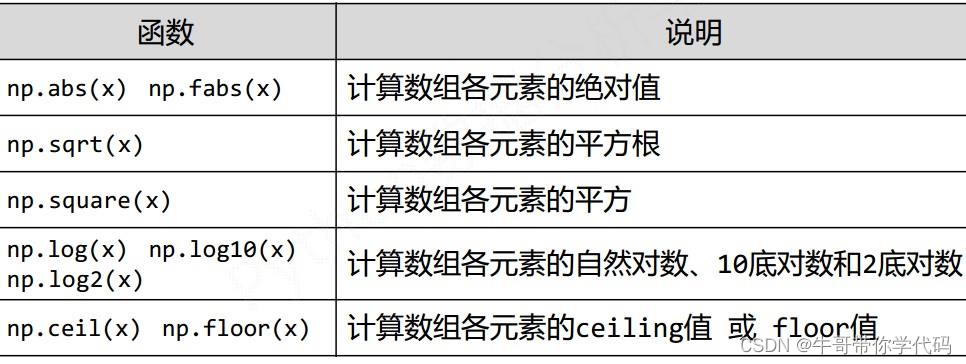ndarray中的数据执行元素级运算的函数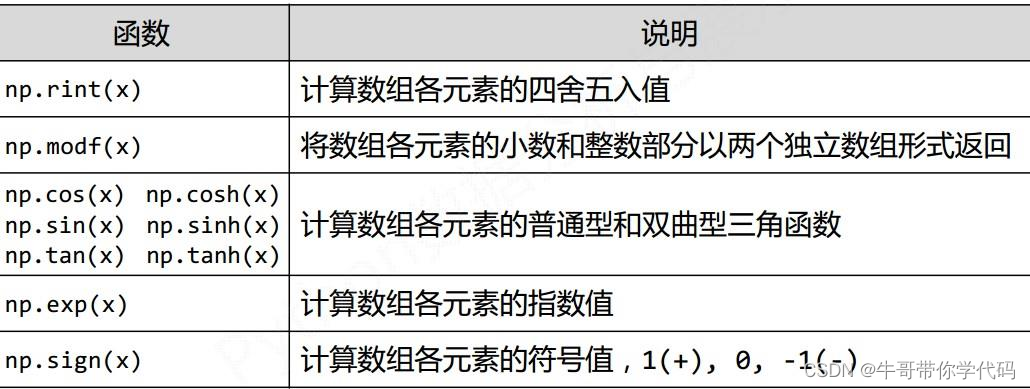``````>>> a=np.arange(1,10).reshape((3,3))
array([[1, 2, 3],
[4, 5, 6],
[7, 8, 9]])
>>> np.sqrt(a)
array([[1. , 1.41421356, 1.73205081],
[2. , 2.23606798, 2.44948974],
[2.64575131, 2.82842712, 3. ]])

>>> np.modf(b)
array([[0., 0.41421356, 0.73205081],
[0. , 0.23606798, 0.44948974],
[0.64575131, 0.82842712, 0. ]]),
array([[1., 1., 1.],[2., 2., 2.],[2., 2.,
3.]]))``````

## 二元算数函数``````>>> np.nodf(b)
>>> a=np.arange(1,10).reshape((3,3))
>>> b=np.sqrt(a)
>>>np.fmax(a,b)
array([[1., 2., 3.],
[4., 5., 6.],
[7., 8., 9.]])

>>> a>b
array([[False,True, True],
[ True, True, True],
[ True, True, True]])``````

## meshgrid函数

xx,yy=np.meshgrid(x,y)

yarray 的转置为列，共 x-dimesion 列的向量。

``````>>> x=np.array([0,1,2,3]) #横坐标
>>> y=np.array([0,1,2,3,4]) #纵坐标
>>> xx,yy=np.meshgrid(x,y)

>>> xx
array([[0,1,2,3],
[0,1,2,3],
[0,1,2,3],
[0,1,2,3],
[0,1,2,3]])

>>> yy
array([[0,0,0,0],
[1,1,1,1],
[2,2,2,2],
[3,3,3,3],
[4,4,4,4])``````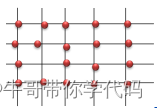## 三元函数——where函数

numpy.where 函数是三元表达式 x if condition else y 的矢量化版本
x if condition else y
||
V
np.where(condition,x,y)

``````>>> arr = np.random.randn(4,4)
>>> arr >0
array([[False, False, False, False],
[ True, True, False, True],
[ True, True, True, False],
[ True, False, True, True]],
dtype=bool)

>>> np.where(arr>0, 2,- 1)
array([[-2, -2, -2, -2],
[ 2, 2, -2 ,2],
[ 2, 2, 2 ,-2],
[ 2, -2, 2 ,2]])``````

## 集合逻辑

NumPy 提供了一些针对一维 ndarray 的基本集合运算，其中 np.unique 用于找出数组中的唯一值并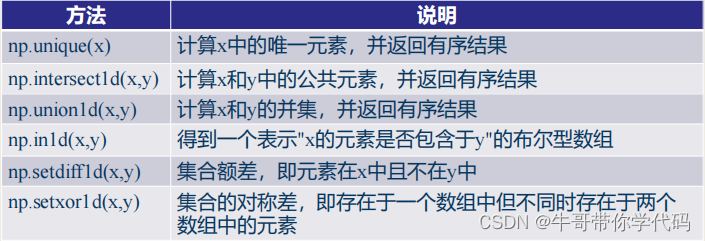## 统计函数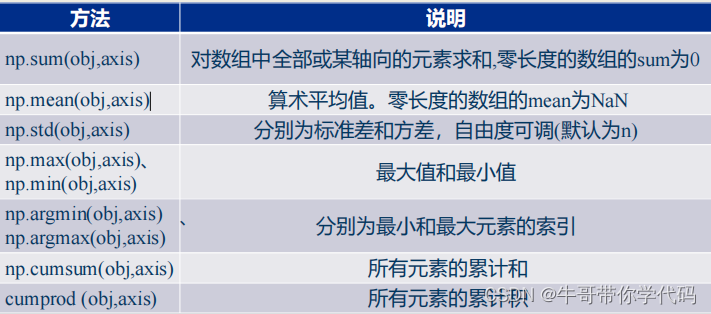## 伪随机数的生成

numpy.random 模块对 Python 内置的 random 进行了补充，增加了一些用于高效生成多种概率分布

Python 内置的 random 模块则只能一次生成一个样本值。
``````>>> from random import normalvariate
>>> N = 1000000
>>> samples = [normalvariate(0, 1) for _ in range(N)]
1.77 s +- 126 ms per loop (mean +- std. dev. of 7 runs, 1 loop each)
>>> np.random.normal(size=N)
61.7 ms +- 1.32 ms per loop (mean +- std. dev. of 7 runs, 10 loops each)``````

## 常用分布函数的随机数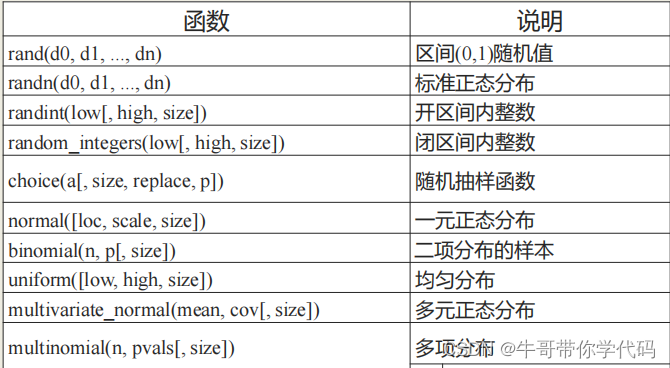## 线性代数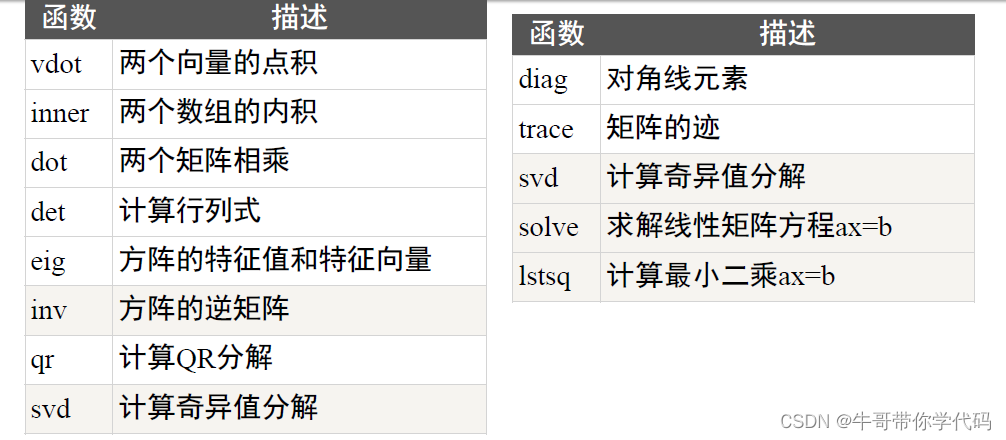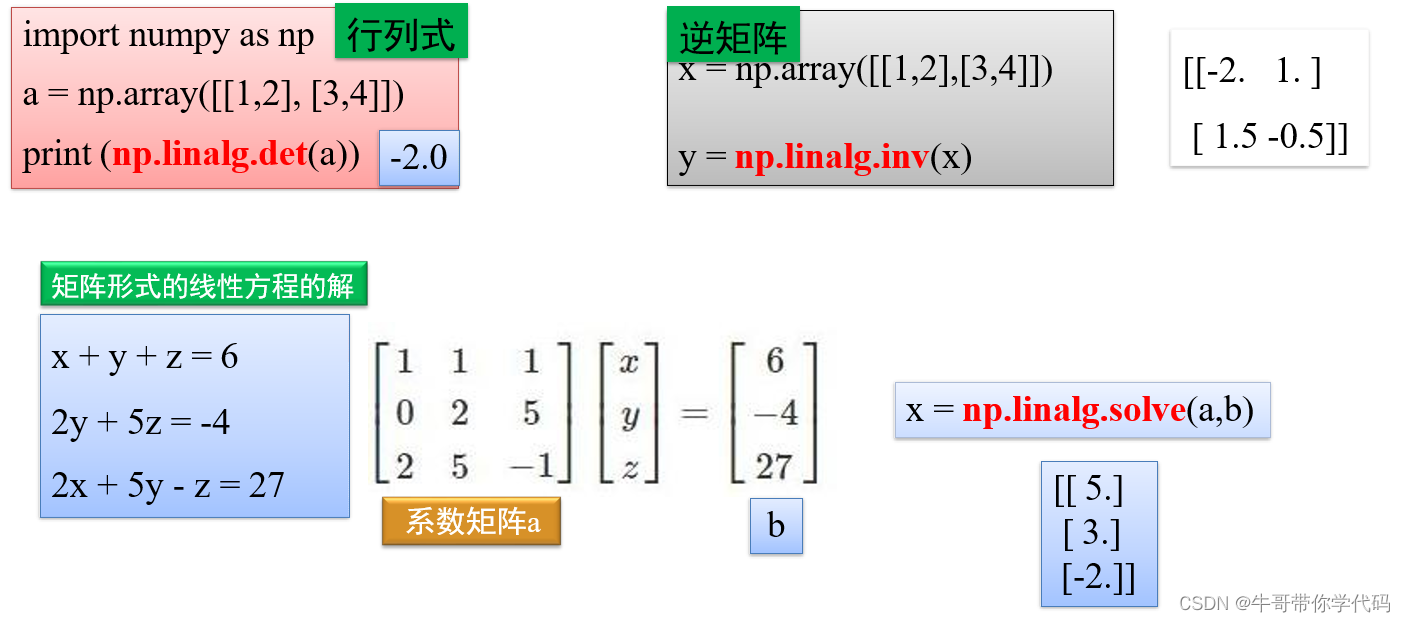## 专用函数

sort 函数返回排序后的数组
argsort 函数返回输入数组排序后的下标；
lexsort 函数根据键值的字典序进行排序；

ndarray 类的 sort 方法可对数组进行原地排序；
msort 函数沿着第一个轴排序；
sort_complex 函数对复数按照先实部后虚部的顺序进行排序增强可维护性

# Numpy文件操作

tofile() fromfile()

.npz 为扩展名。

converters=None, skiprows=0, usecols=None, unpack=False, ndmin=0, encoding='bytes',
max_rows=None,*, like=None )
fname

dtypedelimiter 指定读取文件中数据的分割符， ( 例如 ,csv 文件中的逗号 )
skiprows

converters 对读取的数据进行预处理
usecols

unpack 选择是否将数据进行向量输出
encoding 对读取的文件进行预编码。
``````>>> converters={1:_is_num}
#字典的形式，对指定 的列执行相关操作``````

## 读文件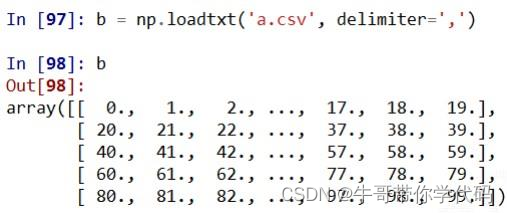## 写文件

np.savetxt(frame, array, fmt='%.18e', delimiter=None)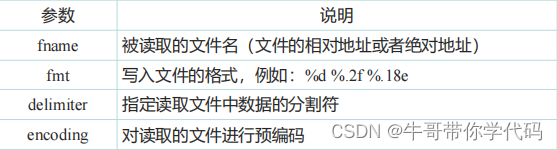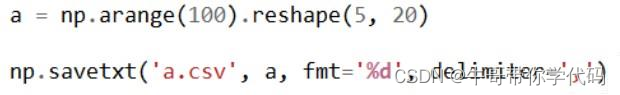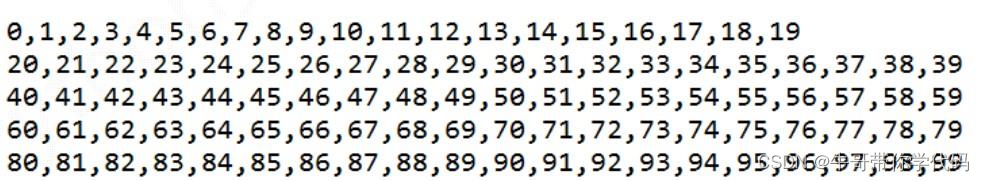##传送门：Python全套学习笔记

### 关于产品MVP的定义与实践

MVP是什么&#xff1f; MVP&#xff08;minimum viable product&#xff0c;最小化可行产品&#xff09;概念最早由埃里克莱斯提出&#xff0c;刊载于哈弗商业评论&#xff0c;并有出版物《精益创业》。 作用&#xff1a; MVP是进行一种小样调研&#xff0c;快速进入市场、接触…

### 用 AWTK 和 AWPLC 快速开发嵌入式应用程序 (3)- 定时器

AWPLC 目前还处于开发阶段的早期&#xff0c;写这个系列文章的目的&#xff0c;除了用来验证目前所做的工作外&#xff0c;还希望得到大家的指点和反馈。如果您有任何疑问和建议&#xff0c;请在评论区留言。 1. 背景 AWTK 全称 Toolkit AnyWhere&#xff0c;是 ZLG 开发的开源…

### 项目实战——配置MySQL与Spring Security模块

PS&#xff1a;本篇文章主体内容并不涉及复杂代码的编写~~请大家放心食用hh 目录 一、整体框架 二、配置MySQL 1、下载MySQL 2、安装MySQL 3、配置MySQL 4、IDEA 连接 MySQL 三、配置 SpirngBoot 1、添加依赖 2、在 application.properties 中添加数据库配置 3、实现简单…

### RHCE学习 --- 第五次作业

RHCE学习 — 第五次作业 1&#xff0c;首先在服务器端创建相应目录 2&#xff0c;修改配置文件 3&#xff0c;在两个目录下分别创建文件以供测试 4&#xff0c;重启服务使配置生效 5&#xff0c;在服务器端查看共享的目录资源 注意&#xff1a;记得关闭服务器端的防火墙的selin…